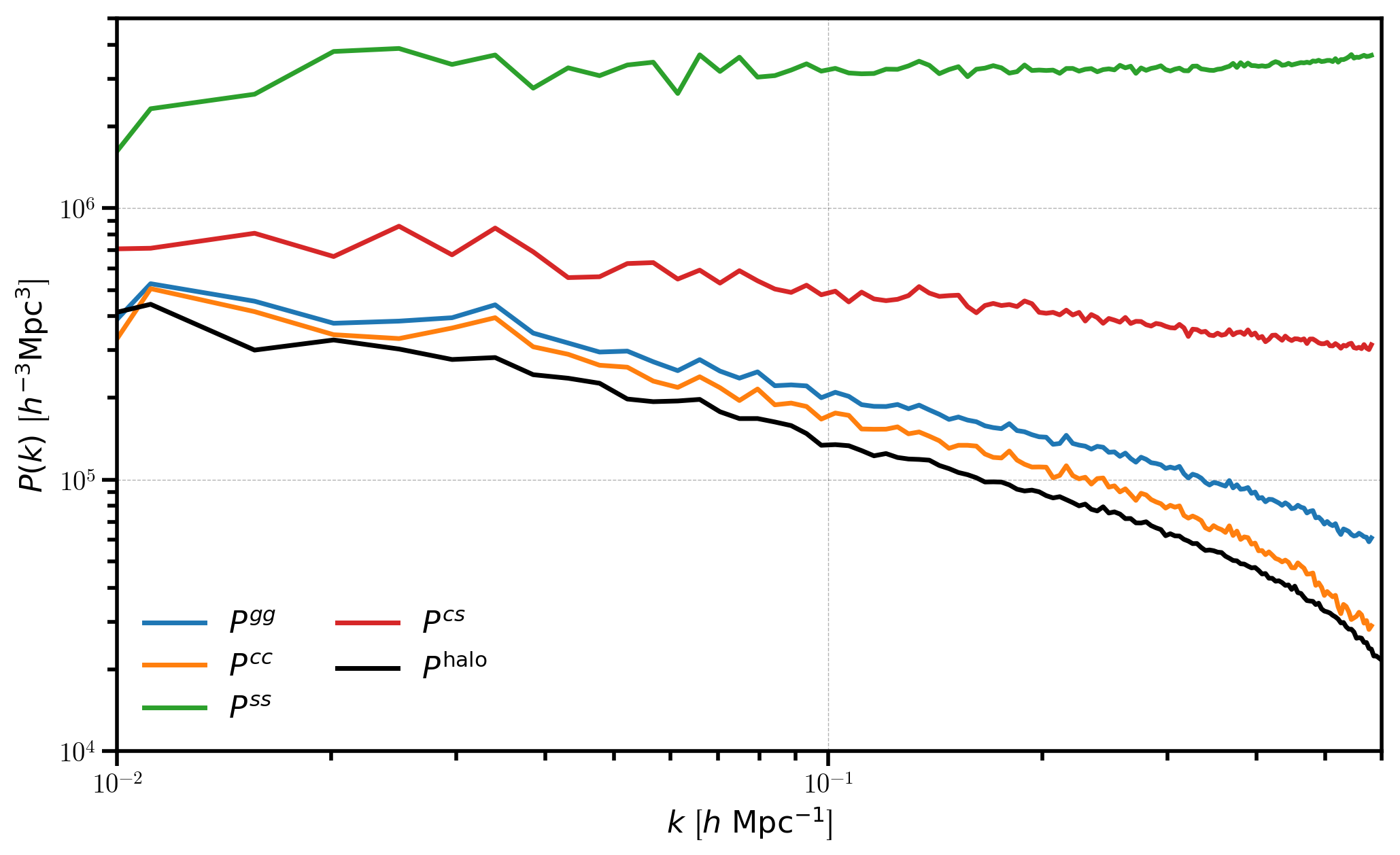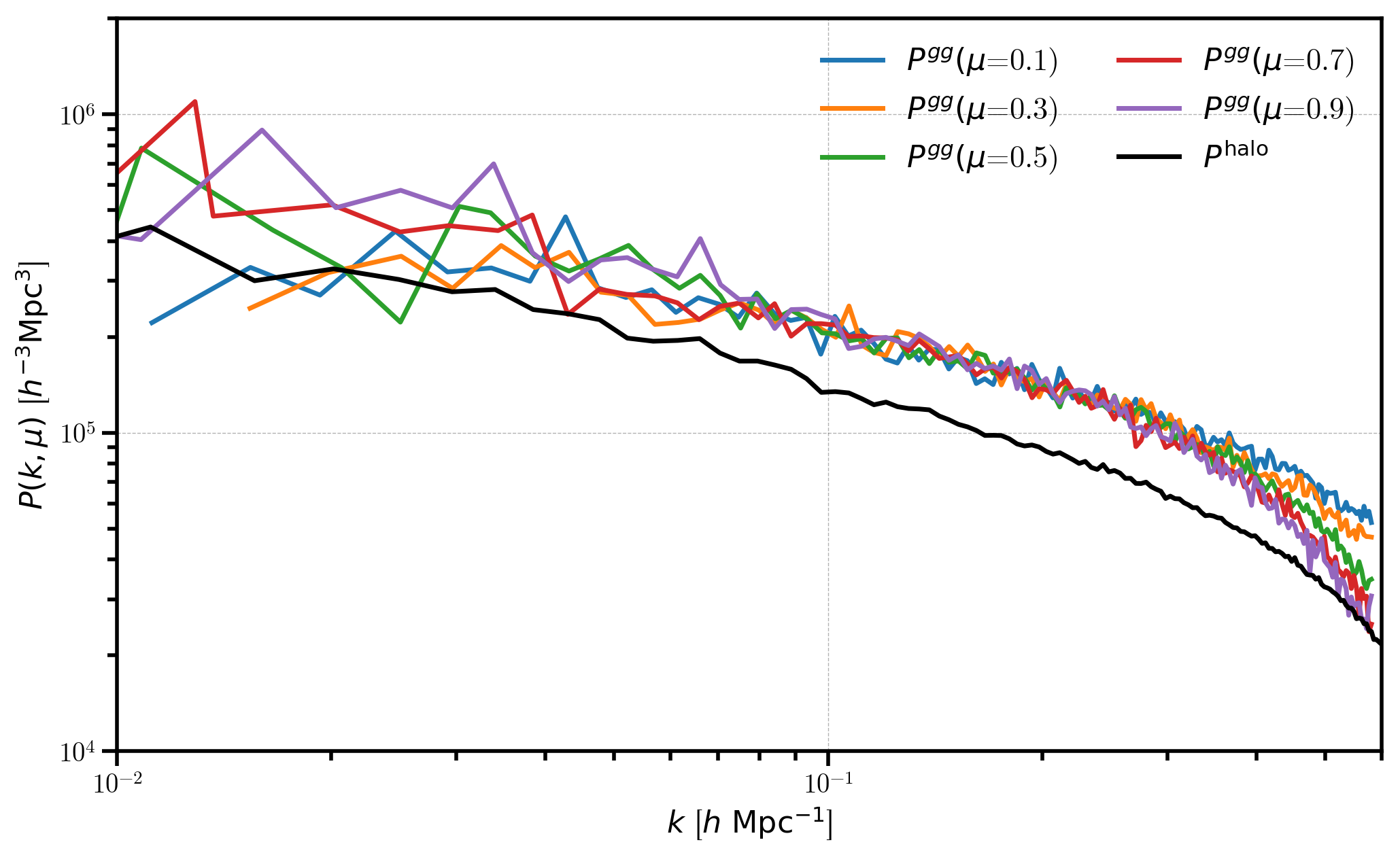# Halo Occupation Distribution (HOD) Mocks¶

In this notebook, we will generate a mock halo catalog by running a FOF finder on a LogNormalCatalog. We will then populate those halos with galaxies via the HOD technique and compare the galaxy and halo power spectra in both real and redshift space.

Note

When viewing results in this notebook, keep in mind that the FOF catalog generated from the LogNormalCatalog object is only semi-realistic. We choose to use the LogNormalCatalog rather than a more realistic data set due to the ease with which we can load the mock data.

:

%matplotlib inline
%config InlineBackend.figure_format = 'retina'

:

from nbodykit.lab import *
from nbodykit import setup_logging, style

import matplotlib.pyplot as plt
plt.style.use(style.notebook)

:

setup_logging() # turn on logging to screen


## Generating Halos via the FOF Algorithm¶

We’ll start by generating a mock galaxy catalog at $$z=0.55$$ from a log-normal density field.

:

redshift = 0.55
cosmo = cosmology.Planck15
Plin = cosmology.LinearPower(cosmo, redshift, transfer='EisensteinHu')
b1 = 2.0

input_cat = LogNormalCatalog(Plin=Plin, nbar=3e-3, BoxSize=1380., Nmesh=256, bias=b1, seed=42)


And then, we run a friends-of-friends (FOF) algorithm on the mock galaxies to identify halos, using a linking length of 0.2 times the mean separation between objects. Here, we only keep halos with at least 5 particle members.

:

fof = FOF(input_cat, linking_length=0.2, nmin=5)


Now, we set the particle mass as $$10^{12} \ M_\odot/h$$ and create a HaloCatalog using the Planck 2015 parameters at a redshift of $$z = 0.55$$. Here, ‘vir’ specifies that we wish to create the Mass column as the virial mass. This mass definition is necessary for computing the analytic concentration, needed when generating the HOD galaxies.

:

halos = fof.to_halos(cosmo=cosmo, redshift=redshift, particle_mass=1e12, mdef='vir')


Finally, we convert the HaloCatalog object to a format recognized by the halotools package and initialze our HOD catalog, using the default HOD parameters.

:

halotools_halos = halos.to_halotools()
hod = HODCatalog(halotools_halos)

[ 000048.48 ]   0: 10-30 20:53  HOD             INFO     satellite fraction: 0.05
[ 000048.48 ]   0: 10-30 20:53  HOD             INFO     mean log10 halo mass: 13.10
[ 000048.49 ]   0: 10-30 20:53  HOD             INFO     std log10 halo mass: 0.31


Using the gal_type column, we can create subsets of the HOD catalog that contain only centrals and only satellites.

:

cen_idx = hod['gal_type'] == 0
sat_idx = hod['gal_type'] == 1

cens = hod[cen_idx]
sats = hod[sat_idx]


## Real-space Power Spectra¶

In this section, we compute the real-space power spectrum of the FOF halos and the HOD galaxies and compare.

:

# compute P(k) for the FOF halos
halo_power = FFTPower(halos, mode='1d', Nmesh=512)

[ 000049.20 ]   0: 10-30 20:53  CatalogMesh     INFO     painted 24720 out of 24720 objects to mesh
[ 000049.20 ]   0: 10-30 20:53  CatalogMesh     INFO     mean particles per cell is 0.000184178
[ 000049.20 ]   0: 10-30 20:53  CatalogMesh     INFO     sum is 24720
[ 000049.20 ]   0: 10-30 20:53  CatalogMesh     INFO     normalized the convention to 1 + delta
[ 000054.30 ]   0: 10-30 20:53  CatalogMesh     INFO     field: (HaloCatalog(size=24720) as CatalogMesh) painting done


And we can compute P(k) for all galaxies, centrals only, satellites only, and the cross power between centrals and satellites.

:

gal_power = FFTPower(hod, mode='1d', Nmesh=256)
cen_power = FFTPower(cens, mode='1d', Nmesh=256)
sat_power = FFTPower(sats, mode='1d', Nmesh=256)
cen_sat_power = FFTPower(cens, second=sats, mode='1d', Nmesh=256)

[ 000063.02 ]   0: 10-30 20:53  CatalogMesh     INFO     painted 9551 out of 9551 objects to mesh
[ 000063.02 ]   0: 10-30 20:53  CatalogMesh     INFO     mean particles per cell is 0.000569284
[ 000063.02 ]   0: 10-30 20:53  CatalogMesh     INFO     sum is 9551
[ 000063.02 ]   0: 10-30 20:53  CatalogMesh     INFO     normalized the convention to 1 + delta
[ 000063.60 ]   0: 10-30 20:53  CatalogMesh     INFO     field: (HODCatalog(logMmin=13.03, sigma_logM=0.38, alpha=0.76, logM0=13.27, logM1=14.08) as CatalogMesh) painting done
[ 000064.60 ]   0: 10-30 20:53  CatalogMesh     INFO     painted 9119 out of 9119 objects to mesh
[ 000064.60 ]   0: 10-30 20:53  CatalogMesh     INFO     mean particles per cell is 0.000543535
[ 000064.60 ]   0: 10-30 20:53  CatalogMesh     INFO     sum is 9119
[ 000064.60 ]   0: 10-30 20:53  CatalogMesh     INFO     normalized the convention to 1 + delta
[ 000065.10 ]   0: 10-30 20:53  CatalogMesh     INFO     field: (HODCatalog(logMmin=13.03, sigma_logM=0.38, alpha=0.76, logM0=13.27, logM1=14.08) as CatalogMesh) painting done
[ 000066.14 ]   0: 10-30 20:53  CatalogMesh     INFO     painted 432 out of 432 objects to mesh
[ 000066.14 ]   0: 10-30 20:53  CatalogMesh     INFO     mean particles per cell is 2.57492e-05
[ 000066.15 ]   0: 10-30 20:53  CatalogMesh     INFO     sum is 432
[ 000066.15 ]   0: 10-30 20:53  CatalogMesh     INFO     normalized the convention to 1 + delta
[ 000067.08 ]   0: 10-30 20:53  CatalogMesh     INFO     field: (HODCatalog(logMmin=13.03, sigma_logM=0.38, alpha=0.76, logM0=13.27, logM1=14.08) as CatalogMesh) painting done
[ 000069.09 ]   0: 10-30 20:53  CatalogMesh     INFO     painted 9119 out of 9119 objects to mesh
[ 000069.09 ]   0: 10-30 20:53  CatalogMesh     INFO     mean particles per cell is 0.000543535
[ 000069.10 ]   0: 10-30 20:53  CatalogMesh     INFO     sum is 9119
[ 000069.10 ]   0: 10-30 20:53  CatalogMesh     INFO     normalized the convention to 1 + delta
[ 000069.93 ]   0: 10-30 20:53  CatalogMesh     INFO     field: (HODCatalog(logMmin=13.03, sigma_logM=0.38, alpha=0.76, logM0=13.27, logM1=14.08) as CatalogMesh) painting done
[ 000070.06 ]   0: 10-30 20:53  CatalogMesh     INFO     painted 432 out of 432 objects to mesh
[ 000070.07 ]   0: 10-30 20:53  CatalogMesh     INFO     mean particles per cell is 2.57492e-05
[ 000070.07 ]   0: 10-30 20:53  CatalogMesh     INFO     sum is 432
[ 000070.07 ]   0: 10-30 20:53  CatalogMesh     INFO     normalized the convention to 1 + delta
[ 000070.74 ]   0: 10-30 20:53  CatalogMesh     INFO     field: (HODCatalog(logMmin=13.03, sigma_logM=0.38, alpha=0.76, logM0=13.27, logM1=14.08) as CatalogMesh) painting done

:

# plot galaxy auto power, centrals auto power, and sats auto power
labels = [r"$P^{gg}$", r"$P^{cc}$", r"$P^{ss}$"]
for i, r in enumerate([gal_power, cen_power, sat_power]):
Pk = r.power
plt.loglog(Pk['k'], Pk['power'].real-Pk.attrs['shotnoise'], label=labels[i])

# central-satellite power
Pk = cen_sat_power.power
plt.loglog(Pk['k'], Pk['power'].real, label=r"$P^{cs}$")

# the halo power
Phalo = halo_power.power
plt.loglog(Phalo['k'], Phalo['power'].real-Phalo.attrs['shotnoise'], c='k', label=r"$P^\mathrm{halo}$")

# add a legend and axis labels
plt.legend(loc=0, ncol=2)
plt.xlabel(r"$k$ [$h \ \mathrm{Mpc}^{-1}$]")
plt.ylabel(r"$P(k)$ [$h^{-3}\mathrm{Mpc}^3$]")
plt.xlim(0.01, 0.6)
plt.ylim(1e4, 5e6)

:

(10000.0, 5000000.0)Here, we see the high bias of the satellite power spectrum (green). Because the satellite fraction is relatively low ($$f_\mathrm{sat} \simeq 0.04$$), the amplitude of the total galaxy power $$P^{gg}$$ is only slightly larger than the centrals auto power $$P^{cc}$$ – the centrals dominate the clustering signal.

## Redshift-space Power Spectra¶

First, we convert HOD galaxy positions from real to redshift space.

:

LOS = [0, 0, 1]
hod['RSDPosition'] = hod['Position'] + hod['VelocityOffset'] * LOS


And then compute $$P(k,\mu)$$ for the full HOD galaxy catalog in five $$\mu$$ bins.

:

mesh = hod.to_mesh(position='RSDPosition', Nmesh=256, compensated=True)
rsd_gal_power = FFTPower(mesh, mode='2d', Nmu=5)

[ 000074.18 ]   0: 10-30 20:53  CatalogMesh     INFO     painted 9551 out of 9551 objects to mesh
[ 000074.18 ]   0: 10-30 20:53  CatalogMesh     INFO     mean particles per cell is 0.000569284
[ 000074.18 ]   0: 10-30 20:53  CatalogMesh     INFO     sum is 9551
[ 000074.18 ]   0: 10-30 20:53  CatalogMesh     INFO     normalized the convention to 1 + delta
[ 000074.77 ]   0: 10-30 20:53  CatalogMesh     INFO     field: (HODCatalog(logMmin=13.03, sigma_logM=0.38, alpha=0.76, logM0=13.27, logM1=14.08) as CatalogMesh) painting done


Finally, plot the galaxy $$P(k,\mu)$$ and compare to the measured halo power in real space.

:

pkmu = rsd_gal_power.power

# plot each mu bin
for i in range(pkmu.shape):
Pk = pkmu[:,i]
label = r'$P^{gg}(\mu$=%.1f)' %pkmu.coords['mu'][i]
plt.loglog(Pk['k'], Pk['power'].real - Pk.attrs['shotnoise'], label=label)

# the halo power
Phalo = halo_power.power
plt.loglog(Phalo['k'], Phalo['power'].real-Phalo.attrs['shotnoise'], c='k', label=r"$P^\mathrm{halo}$")

# add a legend and axis labels
plt.legend(loc=0, ncol=2)
plt.xlabel(r"$k$ [$h \ \mathrm{Mpc}^{-1}$]")
plt.ylabel(r"$P(k, \mu)$ [$h^{-3}\mathrm{Mpc}^3$]")
plt.xlim(0.01, 0.6)
plt.ylim(1e4, 2e6)

:

(10000.0, 2000000.0)In this figure, we see the effects of RSD on the HOD catalog, with the power spectrum damped at higher $$\mu$$ on small scales (larger $$k$$).